Next: Implementation of a wave Up: Wave propagation in an Previous: A first reservoir flow

# Seismic wave simulator

Seismic experiments complement well measurements. Seismic surveys record the continuous wavefield generated by impulsive sources and reflected or transmitted by geological subsurface layers. Traditionally, the recorded data is processed to reveal the structure of the three-dimensional subsurface geology. In the past decade, geophysicists developed additional seismic processing methods to estimate concrete subsurface rock properties, such as the ratio of compressional and shear wave velocity or bulk modulo or even density (). Recently, geophysicists employ repeated seismic surveys over an actively exploited field to estimate changes in the reservoir's fluid contents. Assuming that the reservoir rock is unchanged, such time-lapse or 4-D experiments relate subtle wavefield differences to reservoir fluid changes. Unfortunately, seismic surveys themselves are complex field experiments that are difficult to repeat exactly. Variations in recording geometry, instrumentation, and wave source may lead to wavefield variations that are falsely interpreted as changes in reservoir fluid contents.

Wave equation. For this study, I chose Gassmann's formulation of the wave equation for porous rock. Gassmann's formulation resembles the formulation for homogeneous rocks and is widely used in rock physics studies. Furthermore, Gassmann's formulation shares a parameterization similiar to the parameterization for reservoir fluid flow simulations.

The generic wave equation for an isotropic, linear, elastic medium is(9)
where K is the materials bulk modulo andis its shear modulo. This equation approximates the wave propagation in many non-isotropic, non-linear, and non-elastic materials if the heterogenities of the material and the ampltiude of the wave are small compared to the wave's wavelength.

The solutions to the isotropic wave equation can be separated into a compressional mode (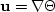) and a shear mode (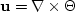). Substitution of these two modes into the isotropic wave equation 9 yields the same simplified wave equation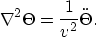(10)
but with two distinct distinct propagation velocities v = vs and v = vp. In terms of the material parameters of the isotropic wave equation the compressional wave velocity is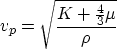(11)
and the shear wave velocity is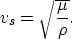(12)
Gassmann show's that these equations for homogeneous isotropic material approximate the wave propagation in porous rocks if the material parameters are the appropriate averages of the rock's constituents.

The density of the porous rock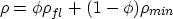(13)
is the average of the fluid density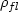and the rock matrix density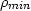weighted by the porosity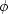. In the case of oil, water, and gas - the petroleum engineer's black oil model - the fluid density is the average of the density of the various components weighted by their saturation: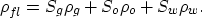(14)

According to Gassmann, the effective bulk modulo of the saturated porous rock Ks is a weighted average of the bulk modulo of the dry rock skeleton Kd, the bulk modulo of the constituent mineral material Km, and the bulk modulo of the saturating fluid Kf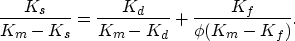(15)
Isolating the unknown Ks yieldswhere b = Kd / Km. The bulk modulo of an oil-water-gas fluid mixture is the average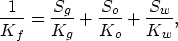(16)
where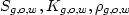are the saturations, bulk moduli, and densities of gas, oil, and water phases.

Finally, the shear moduli of the saturated rock is unchanged by the fluid pore contents(17)

The expressions satisfy the limiting cases. A solid medium impliesand Kf = Ks = Km. A fluid medium implies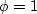and Km = 0 and Ks = Kf. Gassmann's expressions approximate a porous rock only at low frequencies and is strictly valid only in the static (zero-frequency) case. The given averages only approximate the properties of a saturated rock, if the phases in the computational cell are mixed uniformly at very small scale.

Problems and hope for formulation The formulation of a porous rock model does not guarantee its applicability in practice. Traditional AVO studies have shown that seismic reflection experiments are rather insensitive to density changes. Furthermore, the drastic amplitude effect of gas saturation is insensitive to the amount of gas: large and small gas saturations cause almost the same bright spots in seismic sections (). In general, traditional seismic surface experiments resolved little beyond reflectivity and compressional wave velocity. However, the innovation of time-lapse experiments gives seismic experiments a particular sensitivity to saturation changes. However, the optimal formulation of the simulation and inversion problem is still unclear.Next: Implementation of a wave Up: Wave propagation in an Previous: A first reservoir flow
Stanford Exploration Project
3/9/1999